JMSLTM Numerical Library 7.2.0
com.imsl.math

## Interface FeynmanKac.Boundaries

• Enclosing class:
FeynmanKac

`public static interface FeynmanKac.Boundaries`
Public interface for user supplied boundary coefficients and terminal condition the PDE must satisfy.
• ### Method Summary

Methods
Modifier and Type Method and Description
`void` ```leftBoundaries(double time, double[][] coefficients)```
Returns the coefficient values of the left boundary conditions.
`void` ```rightBoundaries(double time, double[][] coefficients)```
Returns the coefficient values of the right boundary conditions.
`double` `terminal(double z)`
Returns the terminal condition value.
• ### Method Detail

• #### leftBoundaries

```void leftBoundaries(double time,
double[][] coefficients)```
Returns the coefficient values of the left boundary conditions. There are `numLeftBounds` conditions specified at the left end,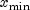. The left boundary conditions are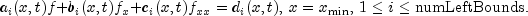Time dependency of the boundary coefficients can be controlled via method `setTimeDependence`. Use of this method will usually yield a more efficient algorithm because some finite element matrices do not have to be reassembled for each `t` value.

Parameters:
`time` - a `double`, the time point at which the boundary coefficients are to be evaluated.
`coefficients` - an output `double` array of dimension `numLeftBounds` by 4 containing the computed boundary coefficient values. The coefficients are stored row-wise according to the matrix scheme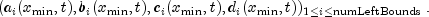• #### rightBoundaries

```void rightBoundaries(double time,
double[][] coefficients)```
Returns the coefficient values of the right boundary conditions. There are `numRightBounds` conditions specified at the right end,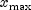. The right boundary conditions are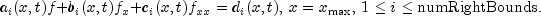Time dependency of the boundary coefficients can be controlled via method `setTimeDependence`. Use of this method will usually yield a more efficient algorithm because some finite element matrices do not have to be reassembled for each `t` value.

Parameters:
`time` - a `double`, the time point at which the boundary coefficients are to be evaluated.
`coefficients` - an output `double` array of dimension `numRightBounds` by 4, containing the computed boundary coefficient values. The coefficients are stored row-wise according to the matrix scheme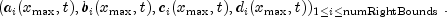• #### terminal

`double terminal(double z)`
Returns the terminal condition value.
Parameters:
`z` - a `double` scalar, the point in `x-` direction, where the terminal condition is evaluated.
Returns:
a `double` scalar, the value of the terminal condition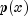at point `z`.
JMSLTM Numerical Library 7.2.0